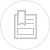# force centrifuge

A solid-liquid suspension is spun in a cylindrical chamber (figure 43) at an angular velocity w (rad · s–1). The average distance separating the particle from the rotation axis is radius R (in m).

A particle having a mass m will be subjected to centrifugal acceleration g which is provided by :

The acceleration generated will always be expressed as a g number with reference to the Earth’s gravitational field :

where P = the weight of the particle,

g = 9.81 m · s–2.

In practice, it is easier to use revolutions per minute-1 as the unit of rotation speed N.

Industrial machines will generate centrifugal fields of 2,000 to 4,000 g in water treatment applications.

Note: force Fs separating the particle from the water (liquid ring) is :

where dS = the particle’s density,

dL = the interstitial liquid’s density.

It is therefore clear that if the particle is subjected to a centrifugal force outside the liquid ring, the forces applied to the particles will be higher.